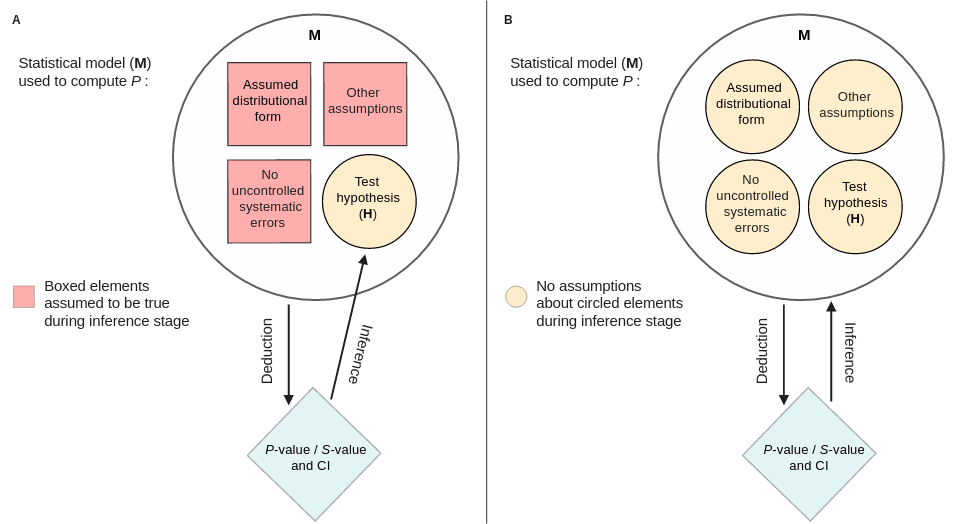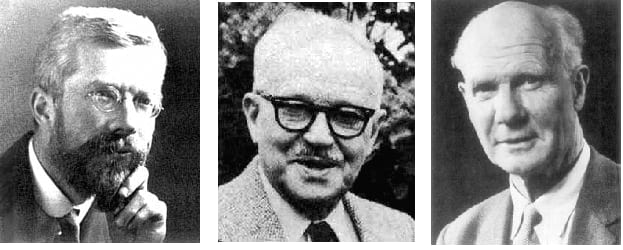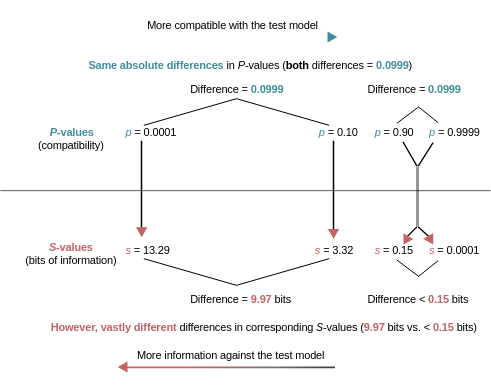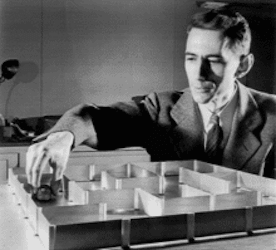# P-Values Are Tough and S-Values Can Help

The P-value doesn’t have many fans. There are those who don’t understand it, often treating it as a measure it’s not, whether that’s a posterior probability, the probability of getting results due to chance alone, or some other bizarre/incorrect interpretation.13

Then there are those who dislike it because they think the concept is too difficult to understand or because they see it as a noisy statistic we’re not interested in.

However, the groups of people mentioned above aren’t mutually exclusive. Many who dislike and criticize the P-value also do not understand its properties and behavior.

## What is a P-value Anyway?

### Definitions

The P-value is the probability of getting a result (specifically, a test statistic) at least as extreme as what was observed if every model assumption, in addition to the targeted test hypothesis (usually a null hypothesis), used to compute it were correct.35

Key assumptions are that randomization was employed (sampling, assignment, etc.), there are no uncontrolled sources of bias (programming errors, equipment defects, sparse-data bias) in the results, and the test hypothesis (often the null hypothesis) is correct. Some of these assumptions can be seen in the figure below from,6 which will be discussed more later below.We assume all those assumptions to be correct (hence, we “condition” on them, even though they are often not correct)6 when calculating the P-value, so that any deviation of the data from what was expected under those assumptions would be purely random error. But in reality such deviations could also be the result of assumptions being false, including but not limited to the test hypothesis. For example, in particle physics, neutrinos were found to be faster than light due to the resulting small test statistic/P-value, but this result was later found to be a result of a loose fiber optic cable that introduced a delay in the timing system.

So the P-value cannot be the probability of one of these assumptions, such as “the probability of getting results due to chance alone.” A statement like this is backwards because it’s quantifying one of the assumptions behind the computations of a P-value.

We assumed this condition to be true (all deviations operating by random error) with several other things, when calculating the P-value, but this does not mean it is actually correct and the calculation of the P-value cannot be the probability of one of those assumptions. It is also worth clarifying that P-values are not probabilities of data, which many like to say to differentiate from probabilities of hypotheses. Rather, P-values are probabilities of “data features”, such as test statistics (i.e. a z-score or $$\chi^{2}$$ statistic) or can be interpreted as the percentile at which the test statistic falls within the expected distribution for the test statistic assuming all the model assumptions are true.7

### Properties

The P-value is a random variable and it’s considered to be valid if it’s well calibrated and meets the validity criterion of being uniform under the null hypothesis of no effect, where every value between 0 and 1 is equally likely (see the histogram below). Many frequentist statisticians do not consider P-values to be useful if they fail to meet this validity criterion, hence they do not recognize variants such as the posterior predictive P-values (which concentrate around values such as 0.5, rather than being uniform) to be valid. This validity criterion can also become a problem in certain scenarios such as adaptive clinical trials with repeated testing, where P-values may no longer become calibrated and require special methods to recalibrate them.## The Different Frameworks Accompanying P-values

The vast majority of researchers interpret the P-value in a dichotomous way such as being statistically significant or not depending on whether or not observed p (the realization of the random variable) falls below a fixed cutoff level (alpha, which is the maximum tolerable type-I error rate).8

This decision-making framework (Neyman-Pearson) may be useful in certain scenarios,9 where some sort of randomization is possible and where there is large control over the experimental conditions, with one of the most notable historical examples being Egon Pearson (son of Karl Pearson and coauthor of Jerzy Neyman) using it to improve quality control in industrial settings.Others choose to interpret the P-value in a Fisherian way,10,11 as a continuous measure of evidence against the very test hypothesis and entire model (all assumptions) used to compute it (let’s go with this for now, even though there are some problems with this interpretation, more on that below).

This interpretation as a continuous measure of evidence against the test hypothesis and the entire model used to compute it can be seen in the figure below from.6 In one framework (left panel), we may assume certain assumptions to be true (“conditioning” on them, i.e, use of random assignment), and in the other (right panel), we question all assumptions, hence the “unconditional” interpretation.The interpretation of the P-value as a continuous measure of evidence against the test model that produced it shouldn’t be confused with other statistics that serve as support measures. Likelihood ratios and Bayes factors are measures of evidence for a model compared to another model.1214

## Compatibilism To The Rescue

The P-value is not a measure of evidence for a model (such as the null/alternative model), it is a continuous measure of the compatibility of the observed data with the model used to compute it.???

If it’s high, it means the observed data are very compatible with the model used to compute it. If it’s very low, then it indicates that the data are not very compatible with the model used to calculate it, and this low value may be due to random variation and/or it may be due to a violation of assumptions (such as the null model not being true, not using randomization, a programming error or equipment defect such as that seen with neutrinos, etc.).

Low compatibility of the data with the model can be implied as evidence against the test hypothesis, if we accept the rest of the model used to compute the P-value. Thus, lower P-values from a Fisherian perspective are seen as stronger evidence against the test hypothesis given the rest of the model.

## Many Criticisms Don’t Hold Up

If we treat the P-value as nothing more or less than a continuous measure of compatibility of the observed data with the model used to compute it (observed p), we won’t run into some of the common misinterpretations such as “the P-value is the probability of a hypothesis”, or the “probability of chance alone”, or “the probability of being incorrect”.3

Thus, many of the “problems” commonly associated with the P-value are not due to the actual statistic itself, but rather researchers’ misinterpretations of what it is and what it means for a study.

The answer to these misconceptions is compatibilism, with less compatibility (smaller P-values) indicating a poor fit between the data and the test model and hence more evidence against the test hypothesis.

A P-value of 0.04 means that assuming that all the assumptions of the model used to compute the P-value are correct, we won’t get data (a test statistic) at least as extreme as what was observed by random variation more than 4% of the time.

To many, such low compatibility between the data and the model may lead them to reject the test hypothesis (the null hypothesis).

### Conceptual Mismatch With Direction

If you recall from above, I wrote that the P-value is seen by many as being a continuous measure of evidence against the test hypothesis and model. Technically speaking, it would be incorrect to define it this way because as the P-value goes up (with the highest value being 1 or 100%), there is less evidence against the test hypothesis since the data are more compatible with the test model. 1 = perfect compatibility of the data with the test model.

As the P-value gets lower (with the lowest value being 0), there is less compatibility between the data and the model, hence more evidence against the test hypothesis used to compute p.

Thus, saying that P-values are measures of evidence against the hypothesis used to compute them is a backward definition. This definition would be correct if higher P-values inferred more evidence against the test hypothesis and vice versa.

### Scaling

Another problem with P-values and their interpretation is scaling. Since the statistic is meant to be a continuous measure of compatibility (and evidence against the test model + hypothesis), we would hope that differences between P-values are equal (on an additive scale), as this makes it easier to interpret.

For example, the difference between 0 and 10 dollars is the same as the difference between 90 and 100 dollars. This makes it easy to think about and compare across various intervals.

Unfortunately, this doesn’t apply to the P-value because it is on the inverse-exponential scale. The difference between 0.01 and 0.10 is not the same as the difference between 0.90 and 0.99.For example, with a normal distribution (above), a z-score of 0 results in a P-value of 1 (perfect compatibility). If we now move to a z-score of 1, the P-value is 0.31. Thus, we saw a dramatic decrease from a P-value of 1 to 0.31 with one z-score. A 0.69 difference in the P-value.

Now let’s go from a z-score of 1 to a z-score of 2. We saw a difference of 0.69 with the change in one z-score before, so the new P-value must be 0.31 - 0.69 = -0.38 right? No. The P-value for a z-score of 2 is 0.045. The P-value for a z-score of 3 is 0.003. Even though we’ve only been moving by one z-score at a time, the changes in P-values don’t remain constant; they become smaller and smaller.

Thus, the difference between the P-values of 0.01 and 0.10 in terms of z-scores is substantially larger than the difference between 0.90 and 0.99.

Again, this makes it difficult to interpret as a statistic across the board, especially as a continuous measure. This can further be seen in the figure from Rafi & Greenland (2020).## S-values as Cognitive Support Aids

The issues described above such as the backward definition and the problem of scaling can make it difficult to conceptualize the P-value as being an evidence measure against the test hypothesis and test model. However, these issues can be addressed by taking the negative log of the P-value $$–\log_{2}(p)$$ , which yields something known as the Shannon information value or surprisal (s) value,6,15 named after Claude Shannon, the father of information theory.16Unlike the P-value, this value is not a probability but rather a continuous measure of information in bits against the test hypothesis and is taken from the observed test statistic computed by the test model.

It also provides a highly intuitive way to think about P-values. Imagine that the variable k is always the nearest integer to the calculated value of s. Now, take for example a P-value of 0.05, the S-value for this would be s = $$–\log_{2}(0.05)$$ which equals 4.3 bits of information embedded in the test statistic, which can be used as evidence against the test hypothesis.

How much evidence is this? k can help us think about this. The nearest integer to 4.3 is 4. Thus, the data which yield a P-value of 0.05 which results in an s value of 4.3 bits of information is no more surprising than getting all heads in 4 fair coin tosses.

Let’s try another example. Let’s say our study gives us a P-value of 0.005, which would indicate to many very low compatibility between the test model and the observed data; this would yield an s value of $$–\log_{2}(0.005) = 7.6$$ bits of information. k which is the closest integer to s would be 8. Thus, the data which yield a P-value of 0.005 are no more surprising than getting all heads on 8 fair coin tosses.

Unlike the P-value, the S-value is more intuitive as a measure that provides evidence against the test hypothesis since its value (information against the test hypothesis) increases with less compatibility, whereas it is the opposite for the P-value.

## Examples

Let’s try using some data to see this in action. I’ll simulate some random data in R from a uniform distribution with the following code,

GroupA <- runif(10, 0, 20)

GroupB <- runif(10, 0, 20)

(RandomData <- data.frame(GroupA, GroupB))
##       GroupA    GroupB
## 1   1.229724  1.451225
## 2   2.427077  1.216582
## 3  17.582448 12.287502
## 4   8.371308  3.663912
## 5   1.106952 18.769553
## 6  17.121398  6.779711
## 7  15.637362  9.674820
## 8  18.633367 10.275540
## 9   2.032834  7.378160
## 10 14.370560 18.405983

We can plot the data and also run an independent samples t-test.Looks interesting. We can obviously see some differences from the graph. Here’s what our test output gives us,

Welch Two Sample t-test

data: GroupA and GroupB

t = 1.358, df = 14.856, p-value = 0.1947

alternative hypothesis: true difference in means is not equal to 0

95 percent confidence interval:

-2.137637   9.627015

sample estimates:

mean of GroupA mean of GroupB

10.258502   6.513812

Okay, we cannot reject the test hypothesis (the null hypothesis) at the 5% level and the confidence interval is ridiculously wide. How can I interpret this P-value of 0.1947 more intuitively?

Let’s convert it into an S-value (here’s a calculator I constructed that converts P-values into S-values).

$–\log_2(0.1947) = 2.36$

S-value= 2.36

That is 2.36 bits of information against the null hypothesis.

How would we interpret it within the context of a given confidence interval? The S-value tells us that values within the computed 95% CI: (-2.13, 9.62) have at most 4.3 bits of information against them.

Remember, k is the nearest integer to the calculated value of s and in this case, would be 2.

So these results (the test statistic) are as surprising as getting all heads in 2 fair coin tosses. Not that surprising.

The S-value is not meant to replace the P-value, and it isn’t superior to the P-value. It is merely a logarithmic transformation of it that rescales it on an additive scale and tells us how much information is embedded in the test statistic and can be used as evidence against the test hypothesis.

It is a useful cognitive device that can help us better interpret the information that we get from a calculated P-value.

I’ve constructed a calculator that converts observed p-values into s-values and provides an intuitive way to think about them.

For a more detailed discussion of S-values, see these articles, in addition to the references below them:


Cole, S. R., Edwards, J. K., and Greenland, S. (2020), “Surprise!,” American Journal of Epidemiology. https://doi.org/10/gg63md.

Rothman, K. J. (2020), “Taken by Surprise,” American Journal of Epidemiology. https://doi.org/10/gg63mf.

Rafi, Z., and Greenland, S. (2020), “Semantic and cognitive tools to aid statistical science: replace confidence and significance by compatibility and surprise,” BMC Medical Research Methodology, Technical Advance, 20, 244. https://doi.org/10.1186/s12874-020-01105-9.

Good, I. J. (1956), “The surprise index for the multivariate normal distribution,” The Annals of Mathematical Statistics, 27, 1130–1135. https://doi.org/10.1214/aoms/1177728079.

Bayarri, M. J., and Berger, J. O. (1999), “Quantifying Surprise in the Data and Model Verification,” *Bayesian Statistics*, 6, 53–82.

Shannon, C. E. (1948), “A mathematical theory of communication,” The Bell System Technical Journal, 27, 379–423. https://doi.org/10.1002/j.1538-7305.1948.tb01338.x.

Greenland, S. (2019), “Valid P-values behave exactly as they should: Some misleading criticisms of P-values and their resolution with S-values,” The American Statistician, 73, 106–114. https://doi.org/10.1080/00031305.2018.1529625.


Acknowledgment: The analogies and concepts in this blog can be attributed to Sander Greenland and his works (many of which are referenced below) and I thank him for his extensive commentary and corrections on several versions of this article.